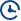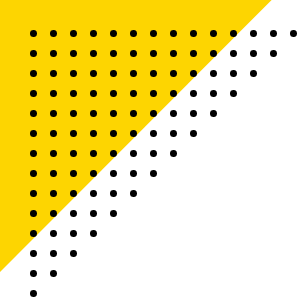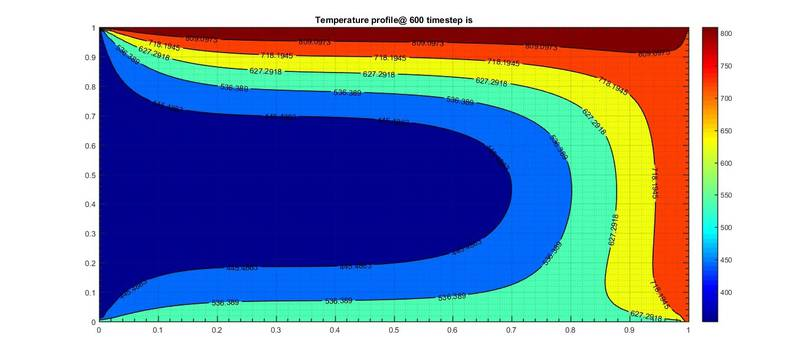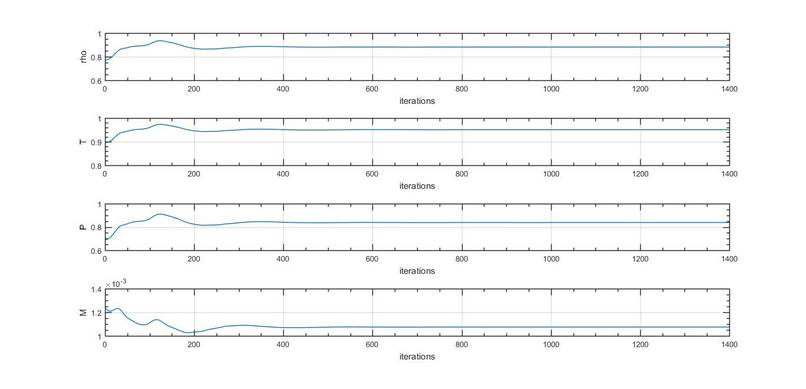# CFD Engineer Master's Certification Program0% EMI Option AvailableDomain : CFD, Thermal ManagementPre-requisites : For Mechanical, Aerospace & Automotive Engineers# OUR RECENT CORPORATE PLACEMENTVimal Raj

CAE Master's Certification ProgramAnand Sanithi

CAE Master's Certification ProgramDilip Kumar S

Master's Certification in BIW Fixture & Plastic DesignRutvik Maasala

CFD Engineer Master's Certification ProgramSaiyan Kumar Behara

CFD Engineer Master's Certification ProgramPuneet Pawar

Master's Certification Program in Hybrid Electric Vehicle Design and AnalysisLuv Kumar

CAE Master's Certification ProgramShashidhar Reddy

Master's Certification Program in Hybrid Electric Vehicle Design and AnalysisHarshal Sukenkar

Master's Certification Program in Hybrid Electric Vehicle Design and AnalysisAshwin Rajesh

Design Engineer Master's Certification ProgramShrey Shah

CFD Engineer Master's Certification ProgramMaster's Certification Program in Hybrid Electric Vehicle Design and AnalysisShikhar Saraswat

Master's Certification Program in Hybrid Electric Vehicle Design and Analysis# Introduction

The Masters in CFD program is a 12 month long, intensive program. The program comprises of 6 courses that train you on all the essential engineering concepts and tools that are essential to get into top OEMs. as a CFD Engineer.
Courses:
1. Introduction to Computational Fluid Dynamics using MATLAB and OpenFOAM
2. Advanced CFD Using ANSYS Fluent
3. Computational Combustion using Python and Cantera
5. IC Engine Calibration using GT-POWER
6. Electronic Cooling Simulations using ANSYS ICEPAK# GET COURSE COUNSELLING TODAY

Get a 1-on-1 demo to understand what is included in the course and how it can benefit you from an experienced sales consultant. The demo session will help you enroll in this course with a clear vision and confidence.

# Course One: Introduction to Computational Fluid Dynamics using MATLAB and OpenFOAM

In this module, you will understand what CFD is and its significance. You’ll also learn what the Navier-Stokes equations are and how they’re derived.

• CFD - An introduction, necessity, advantages, CFD modeling process

• Deriving and understanding the Navier Stokes equations

• Substantial derivative

• Continuity equation

• Momentum equation

• Energy equation

• Significance of Reynold’s number in the NS equations

In this course, you will be writing solvers and getting your hands dirty with different numerical methods. Before we do this, it is very important to understand the essential mathematical and fluid dynamics concepts that you will encounter.

• Basic vector calculus

• Taylor’s series

• Initial and boundary conditions

• Classification of PDEs and their characteristics

• Learning essential Fluid Dynamics quantities and their dimensional analysis

It is essential to establish a rigid foundation before plunging into the farther depths of CFD. This is where you get introduced to MATLAB and learn the basic concepts of CFD by writing MATLAB scripts. Here are some topics that we would cover:

• Getting acclimated to the MATLAB interface

• Numerical discretization and its types

• FDM - understanding different schemes with worked examples in MATLAB

• Deriving own FD schemes using Taylor’s table

• Solving ODEs in MATLAB using the ‘ode45’ solver

In this section, you would venture into the Finite Difference Approach to discretization and solving various benchmark CFD problems in MATLAB. You’ll also be working on two major and two minor projects here. The list of projects are as follows;

• Solving the 1D linear convection equation and performing stability analysis

• Major Project: Simulating 2D unsteady/steady heat conduction equation and studying implicit vs explicit approaches

• Solving coupled linear systems using iterative solvers

• Jacobi

• Gauss-Seidel

• SOR

• Major Project: Simulating Quasi 1D subsonic-supersonic nozzle in FDM and studying conservation vs non-conservation forms of governing equations

OpenFOAM is an open-source toolbox with an in-built numerical solver and pre/post processors for solving CFD Problems. It is based on the Finite Volume Method of discretization. In this section, you will learn how to run a simulation on OpenFOAM and the significance of using an FVM approach. These are the topics you would learn:

• Finite Volume Method and Gauss divergence theorem

• Understanding the Linux environment

• OpenFOAM code organization and case setup

• Detailed blockMeshDict tutorial

It is important to get a real feel of problem-solving using the OpenFOAM software so that you can explore and simulate a wide variety of problems. In this module, we will create a platform that will enable you to start any simulation from scratch.

You will be working on the following major projects.

• Flow over Backward Facing Step

• Code the geometric mesh information inside the C file ‘blockMeshDict’

• Laminar flow through the pipe and Validate results

• Automate the ‘blockMeshDict’ generation on MATLAB

• Characterization of fully developed flow

• Explore different boundary conditions

# Projects Overview

#### 2D Heat Conduction Simulation

In this project, you will learn how to discretize and solve an unsteady and steady diffusion phenomenon using a Finite Difference Method in MATLAB/Octave. Also, you will learn to use both implicit and explicit time integration approaches to solving an unsteady problem. We will work on how to use 3 different iterative methods to solve implicit equations and compare their effectiveness. Finally, you will perform a stability study and understand the criteria to obtain a stable and reliable solution.

• Solve 2D Steady and Transient heat conduction problem

• Implement Jacobi, Gauss-Seidel and Successive Over-Relaxation solvers

• Implement Implicit and Explicit methods to solve the transient part

• Implement Diffusion CFL number-based time step control#### Supersonic Nozzle Flow Simulation using MacCormack Method

In this project, you will simulate the conditions for an inviscid flow inside a Subsonic-Supersonic Convergent-Divergent Isentropic Nozzle. You will perform a quasi-1D simulation using the FDM approach in MATLAB/Octave. The student will then investigate the conservation and non-conservation forms of the governing equations and learn their characteristics and applications.

• 1D supersonic nozzle flow using MacCormack Method

• Implement Conservative and Non-Conservative form

• Implement Courant Number based time step control

• Solve Normalized Governing equations#### Flow over a Backward Facing Step

In this project, you will simulate a laminar viscous flow across a sudden steep expansion in area and study the boundary layer separation phenomena. You will learn how to set up and run a case in OpenFOAM in the Linux environment. You will also learn how to customize the course code to suit this problem. And finally, implement different mesh grading factors and compare the results.

• Simulate this classical CFD benchmarking problem

• Run grid dependency test

• Study the boundary layer separation phenomenon

#### Automated BlockMesh Generation for Meshing Pipe Geometry

In this project, you will simulate the laminar viscous flow through a regular pipe using symmetry and wedge boundary conditions and compare the simulation result with the analytical one obtained using the Hagen-Poiseuille formula. You will to then write a program to automate the generation of the Mesh file. The simulation will be run in OpenFOAM and post-processed in Paraview.

• Automate mesh generation process using MATLAB/Octave

• Perform Wedge Vs. Symmetry BC study

• Understand fully-developed flow and Hydro-dynamic entrance length

• Compare the results with the analytical result from Hagen-Poiseuille formula

# Course Two: Advanced CFD Using ANSYS Fluent

In this module, you will learn about CFD and its uses. You will also be introduced to the basic governing equations solved and many schemes and algorithms used to stabilize and improve the accuracy of the solution.

• Governing equations of fluid motion

• Numerical discretization

• Fluid solver

• Boundary conditions

• Post-processing

In this module, the focus is to simulate basic compressible and incompressible flows using ANSYS Fluent.You will be introduced to the streamlined workflow on the Workbench tool from geometry creation to the solution post-processing procedure.

You will be getting hands-on experience in

• Geometry creation

• Meshing

• Boundary and initial condition calculation

• Setting up solution algorithms

• Solving and post-processing

In this module, the focus is to simulate basic compressible and incompressible steady-state simulations. This provides you an introduction to the solution setup procedure for a steady-state simulation.

You will get hands-on experience in

• Geometry creating using space claim

• How to setup steady-state simulations?

• Checking for convergence and understanding when the simulation converges for different Boundary Conditions?

• How to create runtime animation of engineering parameters?

• Project 1 - HVAC simulation inside a mixing TEE

• Project 2 - Performing a parametric study on flow inside a gate valve

• Project 3 - Performance characterization of a cyclone separator

Meshing is an important component in CFD analysis. Improper meshing can lead to bad results. In this module, you will learn the different meshing techniques that can improve the solution accuracy with a balanced computational cost.

• Methods of providing local refinement like a sphere of influence, body sizing, etc.

• Concept of Y plus and its importance

• Inflation layers and controls

• Mesh dependence test